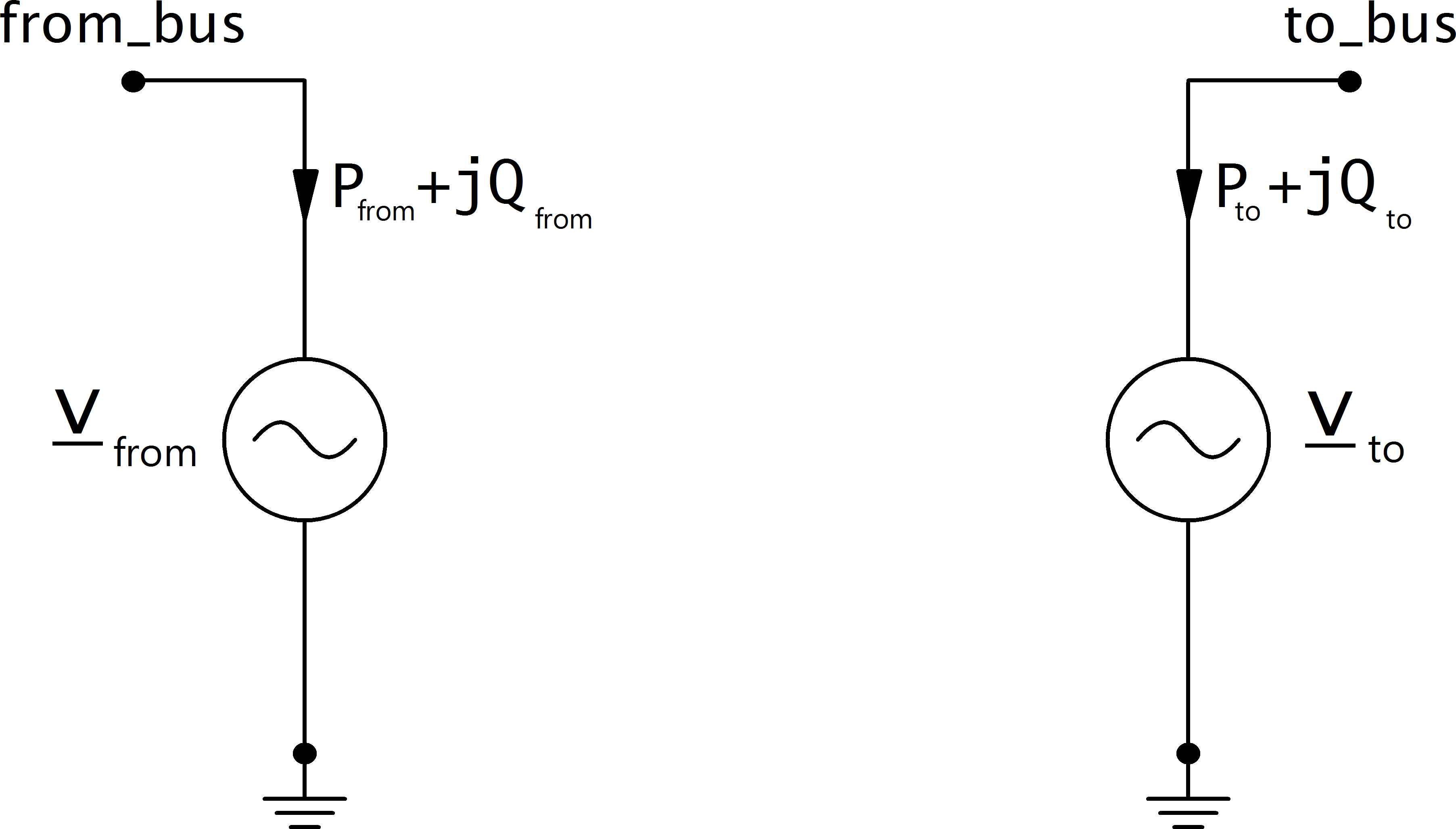DC Line¶

Create Function¶

pandapower.create_dcline(net, from_bus, to_bus, p_mw, loss_percent, loss_mw, vm_from_pu, vm_to_pu, index=None, name=None, max_p_mw=nan, min_q_from_mvar=nan, min_q_to_mvar=nan, max_q_from_mvar=nan, max_q_to_mvar=nan, in_service=True)

Creates a dc line.

INPUT:

from_bus (int) - ID of the bus on one side which the line will be connected with

to_bus (int) - ID of the bus on the other side which the line will be connected with

p_mw - (float) Active power transmitted from ‘from_bus’ to ‘to_bus’

loss_percent - (float) Relative transmission loss in percent of active power

transmission

loss_mw - (float) Total transmission loss in kW

vm_from_pu - (float) Voltage setpoint at from bus

vm_to_pu - (float) Voltage setpoint at to bus

OPTIONAL:

index (int, None) - Force a specified ID if it is available. If None, the index one higher than the highest already existing index is selected.

name (str, None) - A custom name for this dc line

in_service (boolean) - True for in_service or False for out of service

max_p_mw - Maximum active power flow. Only respected for OPF

min_q_from_mvar - Minimum reactive power at from bus. Necessary for OPF

min_q_to_mvar - Minimum reactive power at to bus. Necessary for OPF

max_q_from_mvar - Maximum reactive power at from bus. Necessary for OPF

**max_q_to_mvar ** - Maximum reactive power at to bus. Necessary for OPF

OUTPUT:

index (int) - The unique ID of the created element

EXAMPLE:

create_dcline(net, from_bus=0, to_bus=1, p_mw=1e4, loss_percent=1.2, loss_mw=25, vm_from_pu=1.01, vm_to_pu=1.02)

Input Parameters¶

net.dcline

 Parameter Datatype Value Range Explanation name string name of the generator from_bus* integer Index of bus where the dc line starts to_bus* integer Index of bus where the dc line ends p_mw* float $$>$$ 0 Active power transmitted from ‘from_bus’ to ‘to_bus’ loss_percent* float $$>$$ 0 Relative transmission loss in percent of active power transmission loss_mw* float $$>$$ 0 Total transmission loss in MW vm_from_pu* float $$>$$ 0 Voltage setpoint at from bus vm_to_pu* float $$>$$ 0 Voltage setpoint at to bus max_p_mw** float $$>$$ 0 Maximum active power transmission min_q_from_mvar** float Minimum reactive power at from bus max_q_from_mvar** float Maximum reactive power at from bus min_q_to_mvar** float Minimum reactive power at to bus max_q_to_mvar** float Maximum reactive power at to bus in_service* bool True/False specifies if DC line is in service

*necessary for executing a power flow calculation
**optimal power flow parameter

Note

DC line is only able to model one-directional loadflow for now, which is why p_mw / max_p_mw have to be > 0.

Electric Model¶

A DC line is modelled as two generators in the loadflow:The active power at the from side is defined by the parameters in the dcline table. The active power at the to side is equal to the active power on the from side minus the losses of the DC line.

\begin{align*} P_{from} &= p\_mw \\ P_{to} &= - (p\_mw - loss\_mw) \cdot (1 - \frac{loss\_percent}{100}) \end{align*}

The voltage control with reactive power works just as described for the generator model. Maximum and Minimum reactive power limits are considered in the OPF, and in the PF if it is run with enforce_q_lims=True.

Result Parameters¶

net.res_dcline

 Parameter Datatype Explanation p_from_mw float active power flow into the line at ‘from_bus’ [MW] q_from_mvar float reactive power flow into the line at ‘from_bus’ [kVar] p_to_mw float active power flow into the line at ‘to_bus’ [MW] q_to_mvar float reactive power flow into the line at ‘to_bus’ [kVar] pl_mw float active power losses of the line [MW] vm_from_pu float voltage magnitude at ‘from_bus’ [p.u] va_from_degree float voltage angle at ‘from_bus’ [degree] vm_to_pu float voltage magnitude at ‘to_bus’ [p.u] va_to_degree float voltage angle at ‘to_bus’ [degree]
\begin{align*} p\_from\_mw &= P_{from} \\ p\_to\_mw &= P_{to} \\ pl\_mw &= p\_from\_mw + p\_to\_mw \\ q\_from\_mvar &= Q_{from} \\ q\_to\_mvar &= Q_{to} \\ va\_from\_degree &= \angle \underline{v}_{from} \\ va\_to\_degree &= \angle \underline{v}_{to} \\ vm\_from\_degree &= |\underline{v}_{from}| \\ vm\_to\_degree &= |\underline{v}_{to}| \\ \end{align*}